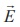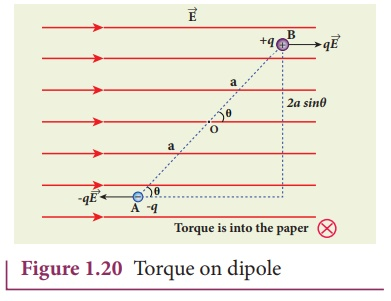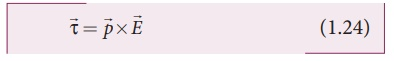Home | | Physics 12th Std | Torque experienced by an electric dipole in the uniform electric field

# Torque experienced by an electric dipole in the uniform electric field

Torque experienced by an electric dipole in the uniform electric field

Torque experienced by an electric dipole in the uniform electric field

Consider an electric dipole of dipole momentplaced in a uniform electric fieldwhose field lines are equally spaced and point in the same direction. The charge +q will experience a forcein the direction of the field and charge –q will experience a force -qin a direction opposite to the field. Since the external fieldis uniform, the total force acting on the dipole is zero. These two forces acting at different points will constitute a couple and the dipole experience a torque as shown in Figure 1.20. This torque tends to rotate the dipole. (Note that electric field lines of a uniform field are equally spaced and point in the same direction).

The total torque on the dipole about the point OUsing right-hand corkscrew rule (Refer XI, volume 1, unit 2), it is found that total torque is perpendicular to the plane of the paper and is directed into it.The    magnitude of the total torquewhere θ is the angle made bywith. Since p = 2aq, the torque is written in terms of the vector product asThe magnitude of this torque is τ = pE sinθ and is maximum when θ = 90 .

This torque tends to rotate the dipole and align it with the electric field. Onceis aligned with, the total torque on the dipole becomes zero.

If the electric field is not uniform, then the force experienced by +q is different from that experienced by –q. In addition to the torque, there will be net force acting on the dipole. This is shown in Figure 1.21.EXAMPLE 1.11

A sample of HCl gas is placed in a uniform electric field of magnitude 3 × 104 N C-1. The dipole moment of each HCl molecule is 3.4 × 10-30 Cm. Calculate the maximum torque experienced by each HCl molecule.

Solution

The maximum torque experienced by the dipole is when it is aligned perpendicular to the applied field.

τmax = pE sin 90 = 3 .4×10−30 ×3 ×104 N m

τmax = 10.2 ×10−26 N m

Study Material, Lecturing Notes, Assignment, Reference, Wiki description explanation, brief detail
12th Physics : Electrostatics : Torque experienced by an electric dipole in the uniform electric field |